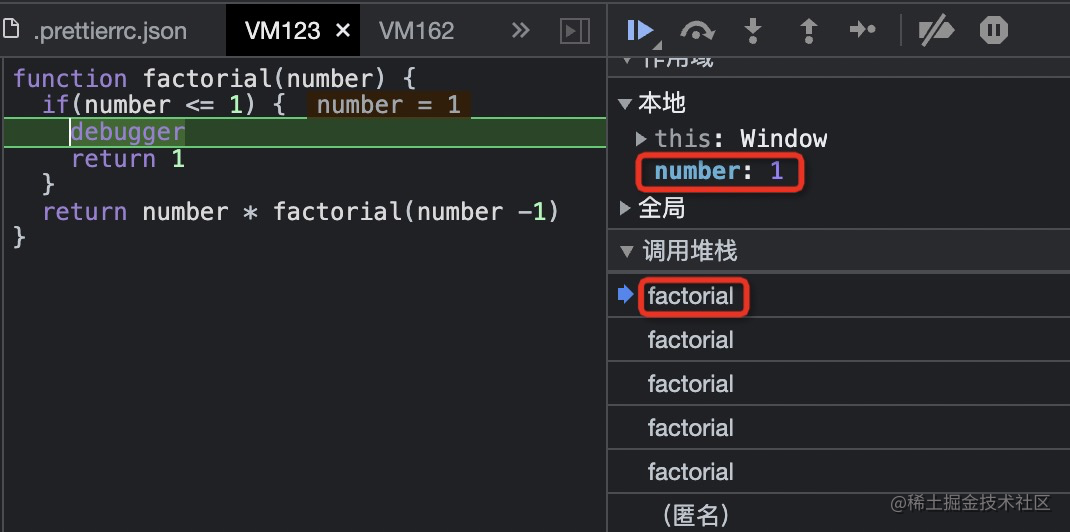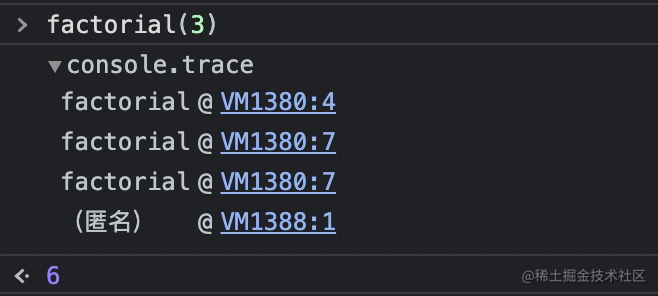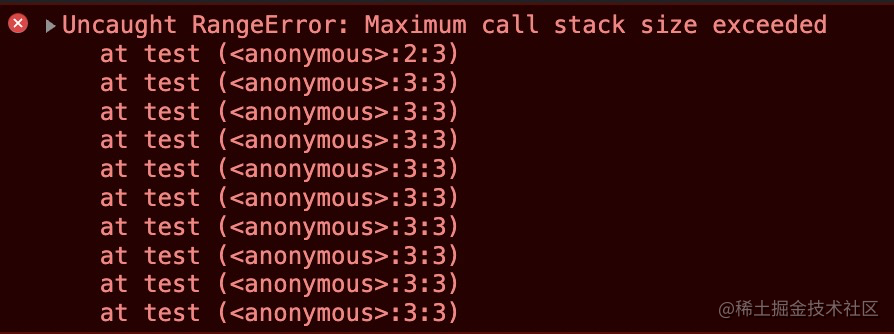# 认识递归

## 什么是递归

• 函数调用自身
• 两个函数互相调用

```// 调用自身function recursiveFun(someParam) {  recursiveFun(someParam);}
// 两个函数互相调用function recursiveFun1(someParam) {  recursiveFun2(someParam);}function recursiveFun2(someParam) {  recursiveFun1(someParam);}```

`function recursiveFun(someParam) {  let is_finish = someParam[xx] == 'xxx';  if (is_finish) {    return true; // 终止循环  }  recursiveFun(someParam);}`

## 计算一个数的阶乘

`n` 的阶乘，定义为 `n!`，表示从 1 到 n 的整数的乘积。

`n * (n-1) * (n-2) * ... * 1`

`function recursiveFun(number) {  if (number <= 0) return undefined;  let total = 1;  for (let n = number; n > 1; n--) {    total = total * n;  }  return total;}console.log(recursiveFun(5)); // 120`

• factorial(5) = 5 * factorial(4)
• factorial(4) = 4 * factorial(3)
• factorial(n) = n * factorial(n-1) // n>=1

`function factorial(number) {  if (number <= 1) {    return 1;  }  return number * factorial(number - 1);}console.log(factorial(3)); // 6`

## 看清递归的执行顺序

`if (number <= 1) {  debugger;  return 1;}``var count = 1;function test() {  count++;  test();}test();``console.log(count); // 10989`